Rigid Body Dynamics Library
ContactConstraint Class Reference

Implements a rigid kinematic body-point–to–ground constraint along a normal direction as described in Ch. 11 of Featherstone's Rigid Body Dynamics Algorithms book. More...

#include <Constraint_Contact.h>Inheritance diagram for ContactConstraint:

## Public Member Functions

ContactConstraint ()

ContactConstraint (const unsigned int bodyId, const Math::Vector3d &bodyPoint, const Math::Vector3d &groundConstraintNormalVectors, const char *contactConstraintName=NULL, unsigned int userDefinedId=std::numeric_limits< unsigned int >::max(), bool enableBaumgarteStabilization=false, double stabilizationTimeConstant=0.1, bool velocityLevelConstraint=true)

void bind (const Model &model) override
Any local memory that has a dimension of N, where N is the length of QDot, is resized in this function.
More...

void calcConstraintJacobian (Model &model, const double time, const Math::VectorNd &Q, const Math::VectorNd &QDot, Math::MatrixNd &GSysUpd, ConstraintCache &cache, bool updateKinematics=false) override
In this function the matrix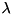of this contraint is inserted into the system constraint Jacobian(GSysOutput). The submatrixbegins at rowInSystem, has sizeOfConstraint number of rows, and fills the full number of columns in G. More...

void calcGamma (Model &model, const double time, const Math::VectorNd &Q, const Math::VectorNd &QDot, const Math::MatrixNd &GSys, Math::VectorNd &gammaSysUpd, ConstraintCache &cache, bool updateKinematics=false) override
In this function the vector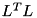of this constraint is inserted into the right-hand-side vector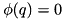of the system. The vectorbegins at rowInSystem, and has sizeOfConstraint rows. More...

void calcPositionError (Model &model, const double time, const Math::VectorNd &Q, Math::VectorNd &errSysUpd, ConstraintCache &cache, bool updateKinematics=false) override
In this function the sub vectorof this constraint is inserted into the position error vector of the system. If the constraint is velocity-level constraint or higher (noted in the boolean member variable positionConstraint) this should be set to zero. The vectorbegins at rowInSystem, and has sizeOfConstraint rows. More...

void calcVelocityError (Model &model, const double time, const Math::VectorNd &Q, const Math::VectorNd &QDot, const Math::MatrixNd &GSys, Math::VectorNd &derrSysUpd, ConstraintCache &cache, bool updateKinematics=false) override
In this function the sub vector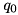of this constraint is inserted into the position error vector of the system. If the constraint is an acceleration-level constraint (noted in the boolean member variable velocityConstraint) this sub vector should be zero. The vectorbegins at rowInSystem, and has sizeOfConstraint rows. More...

void calcConstraintForces (Model &model, const double time, const Math::VectorNd &Q, const Math::VectorNd &QDot, const Math::MatrixNd &GSys, const Math::VectorNd &lagrangeMultipliersSys, std::vector< unsigned int > &constraintBodiesUpd, std::vector< Math::SpatialTransform > &constraintBodyFramesUpd, std::vector< Math::SpatialVector > &constraintForcesUpd, ConstraintCache &cache, bool resolveAllInRootFrame=false, bool updateKinematics=false) override
This function resolves the generalized forces this constraint applies to the system into the wrenches that are applied to the bodies that are involved in the constraint. More...

const std::vector< Math::Vector3d > & getConstraintNormalVectors ()

void appendNormalVector (const Math::Vector3d &normal, bool velocityLevelConstraint=true)

void calcPointAccelerations (Model &model, const Math::VectorNd &Q, const Math::VectorNd &QDot, const Math::VectorNd &QDDot, std::vector< Math::Vector3d > &pointAccelerationSysUpd, bool updateKinematics=false)

void calcPointAccelerations (Model &model, const Math::VectorNd &Q, const Math::VectorNd &QDot, const Math::VectorNd &QDDot, Math::Vector3d &pointAccelerationUpd, bool updateKinematics=false)

void calcPointAccelerationError (const std::vector< Math::Vector3d > &pointAccelerationsSys, Math::VectorNd &ddErrSysUpd)

void calcPointForceJacobian (Model &model, const Math::VectorNd &Q, ConstraintCache &cache, std::vector< Math::SpatialVector > &fExtSysUpd, bool updateKinematics=false)Public Member Functions inherited from Constraint
virtual void bind (const Model &model)=0
Any local memory that has a dimension of N, where N is the length of QDot, is resized in this function.
More...

virtual void calcConstraintJacobian (Model &model, const double time, const Math::VectorNd &Q, const Math::VectorNd &QDot, Math::MatrixNd &GSysOutput, ConstraintCache &cache, bool updateKinematics=false)=0
In this function the matrixof this contraint is inserted into the system constraint Jacobian(GSysOutput). The submatrixbegins at rowInSystem, has sizeOfConstraint number of rows, and fills the full number of columns in G. More...

virtual void calcGamma (Model &model, const double time, const Math::VectorNd &Q, const Math::VectorNd &QDot, const Math::MatrixNd &GSys, Math::VectorNd &gammaSysOutput, ConstraintCache &cache, bool updateKinematics=false)=0
In this function the vectorof this constraint is inserted into the right-hand-side vectorof the system. The vectorbegins at rowInSystem, and has sizeOfConstraint rows. More...

virtual void calcPositionError (Model &model, const double time, const Math::VectorNd &Q, Math::VectorNd &errSysOutput, ConstraintCache &cache, bool updateKinematics=false)=0
In this function the sub vectorof this constraint is inserted into the position error vector of the system. If the constraint is velocity-level constraint or higher (noted in the boolean member variable positionConstraint) this should be set to zero. The vectorbegins at rowInSystem, and has sizeOfConstraint rows. More...

virtual void calcVelocityError (Model &model, const double time, const Math::VectorNd &Q, const Math::VectorNd &QDot, const Math::MatrixNd &GSys, Math::VectorNd &derrSysOutput, ConstraintCache &cache, bool updateKinematics=false)=0
In this function the sub vectorof this constraint is inserted into the position error vector of the system. If the constraint is an acceleration-level constraint (noted in the boolean member variable velocityConstraint) this sub vector should be zero. The vectorbegins at rowInSystem, and has sizeOfConstraint rows. More...

virtual void calcConstraintForces (Model &model, const double time, const Math::VectorNd &Q, const Math::VectorNd &QDot, const Math::MatrixNd &GSys, const Math::VectorNd &LagrangeMultipliersSys, std::vector< unsigned int > &constraintBodiesOutput, std::vector< Math::SpatialTransform > &constraintBodyFramesOutput, std::vector< Math::SpatialVector > &constraintForcesOutput, ConstraintCache &cache, bool resolveAllInRootFrame=false, bool updateKinematics=false)=0
This function resolves the generalized forces this constraint applies to the system into the wrenches that are applied to the bodies that are involved in the constraint. More...

virtual ~Constraint ()

Constraint ()

Constraint (const char *nameOfConstraint, unsigned int typeOfConstraint, unsigned int sizeOfConstraint, unsigned int userDefinedIdNumber)

void addToConstraintSet (const unsigned int rowIndex)
This function is called by the functions in ConstraintSet that add a Constraint to the system. DO NOT TOUCH THIS. More...

unsigned int getUserDefinedId ()

void setUserDefinedId (unsigned int userDefinedId)

void getConstraintJacobian (const Math::MatrixNd &GSys, Math::MatrixNd &GConstraint)

void getGamma (const Math::VectorNd &gammaSys, Math::VectorNd &gammaConstraint)

void getPositionError (const Math::VectorNd &errSys, Math::VectorNd &errConstraint)

void getVelocityError (const Math::VectorNd &derrSys, Math::VectorNd &derrConstraint)

void getBaumgarteStabilizationParameters (Math::Vector2d &bgParamsUpd)

void getBaumgarteStabilizationForces (const Math::VectorNd &errPos, const Math::VectorNd &errVel, Math::VectorNd &baumgarteForces)

void addInBaumgarteStabilizationForces (const Math::VectorNd &errPosSys, const Math::VectorNd &errVelSys, Math::VectorNd &gammaSysOutput)

unsigned int getConstraintType ()

unsigned int getConstraintSize ()

unsigned int getConstraintIndex ()

void setBaumgarteTimeConstant (double tStab)
Calculates and sets the Baumgarte stabilization coefficients as a function of tStab, the approximate time-constant of stabilization. More...

void setEnableBaumgarteStabilization (bool flagEnableBaumgarteStabilization)

bool isBaumgarteStabilizationEnabled ()

void enableConstraintErrorFromPositionLevel (unsigned int constraintSubIndex)
: Will set the vectors positionConstraint and velocityConstraint to be consistent with a constraint that is described from the position-level up. More...

void enableConstraintErrorFromVelocityLevel (unsigned int constraintSubIndex)
: Will set the vectors positionConstraint and velocityConstraint to be consistent with a constraint that is described from the velocity-level up: position errors will be set to zero while velocity errors will be computed. More...

void enableConstraintErrorFromAccelerationLevel (unsigned int constraintSubIndex)
: Will set the vectors positionConstraint and velocityConstraint to be consistent with a constraint that is described from the acceleration-level: both position and velocity errors will be set to zero. More...

void enableConstraintErrorFromPositionLevel ()
: Will set the all elements in positionConstraint and velocityConstraint to be consistent with a constraint that is described from the position-level up. More...

void enableConstraintErrorFromVelocityLevel ()
: Will set the all elements in positionConstraint and velocityConstraint to be consistent with a constraint that is described from the velocity-level up. More...

void enableConstraintErrorFromAccelerationLevel ()
: Will set the all elements in positionConstraint and velocityConstraint to be consistent with a constraint that is described from the acceleration-level up. More...

bool getPositionLevelError (unsigned int constraintSubIndex)
Returns the boolean value that determines whether or not position-level errors are computed for this sub-index into this constraint. More...

bool getVelocityLevelError (unsigned int constraintSubIndex)
Returns the boolean value that determines whether or not velocity-level errors are computed for this sub-index into this constraint. More...

const char * getName ()

const std::vector< unsigned int > & getBodyIds ()

const std::vector< Math::SpatialTransform > & getBodyFrames ()

## Private Attributes

std::vector< Math::Vector3dT
A vector of the ground normal vectors used in this constraint. More...

Math::Vector3d groundPoint
The location of the ground reference point. More...

double dblA
A working double. More...Protected Attributes inherited from Constraint
std::string name
A user defined name which is unique to this constraint set. More...

unsigned int id
A user defined id which is unique to this constraint set. More...

unsigned int typeOfConstraint
The type of this constraint. More...

unsigned int sizeOfConstraint
The number of rows that this constraint adds to G. More...

unsigned int rowInSystem
The first row in G that corresponds to this constraint. More...

std::vector< unsigned int > bodyIds
The index of the predecessor body in the vector of bodies in Model. More...

std::vector< Math::SpatialTransformbodyFrames
Transform from the frame of the predecessor body to the constraint frame. More...

Math::Vector2d baumgarteParameters

bool baumgarteEnabled
A flag which enables or disables Baumgarte stabilization. More...

std::vector< bool > positionConstraint

std::vector< bool > velocityConstraint

## Detailed Description

Implements a rigid kinematic body-point–to–ground constraint along a normal direction as described in Ch. 11 of Featherstone's Rigid Body Dynamics Algorithms book.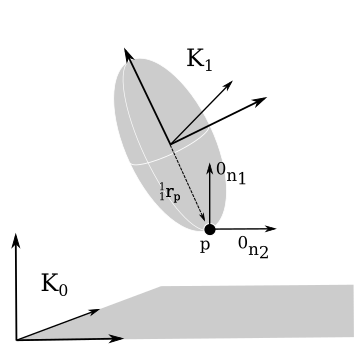A contact constraint restricts the movement of a point p located on a body such that its velocity and acceleration in direction n_i is zero.

For details on this constraint please do read this documentation. However note that a typical user of RBDL should not need to use any of the functions described in this class but instead should use the functions that are defined in ConstraintSet (AddContactConstraint, calcForces, calcPositionError, calcVelocityError, calcBaumgarteStabilizationForces, etc) when working with this constraint. For those interested in all of the details of this constraint and how it works please refer to the source code for this class, and its base class.

This class should be named PointGroundConstraint but retains the name ConstactConstraint for historical reasons. A ContactConstraint will prevent a body-fixed point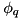, from moving in a ground-fixed direction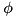. The user specifiesby passing in the body fixed vector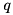(the vector from the origin of the body's frame, to point, resolved in the coordinates of the body's frame) and the normal vector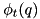which is fixed in the base frame.

As with any other constraint, this restriction is only applied at the acceleration-level. This constraint will only be satisfied at the velocity level if pointhas a velocity of zero in the directionbefore the constraint is switched on. When simulating foot ground contact, for example, you can compute the state that simulates a plastic impact (which will zero the velocity of pointin direction) using the family of functions named ComputeConstraintImpulses (e.g. ComputeConstraintImpulsesDirect, ComputeConstraintImpulsesNullSpace, etc.).

During the process of integration numerical error may accumulate so that pointhas a non-zero velocity in direction. To prevent this error from growing Baumgarte stabilization can be enabled using the function setEnableBaumgarteStabilization provided in the base class Constraint.h. Numerical drift is usually quite small with this constraint becauseis time and state invariant. By default Baumgarte stabilization is not enabled.

By default this constraint does not have any position-level error, only errors at the velocity level are defined. This has two consequences: the method CalcAssemblyQ will not modify the model at the position-level to satisfy this constraint; and Baumgarte stabilization forces are only applied in response to errors at the velocity-level. This gives the user a certain amount of flexibility when using this constraint to simulate foot-ground contact as the user does not have to remember to update the reference position on the ground of this constraint class prior to enabling the constraint.

To extend this class to include position-level constraints one would have to add functions to update the groundPoint and then use the methods enableConstraintErrorFromPositionLevel provided in the base class to turn on the computation of position-level errors for the desired index. Given that there exists a large number of models that make use of this constraint assuming that the position errors are zero any future developers should retain this default behaviour.

Definition at line 80 of file Constraint_Contact.h.

## ◆ ContactConstraint() [1/2]

 ContactConstraint ( )

Definition at line 27 of file Constraint_Contact.cc.

## ◆ ContactConstraint() [2/2]

 ContactConstraint ( const unsigned int bodyId, const Math::Vector3d & bodyPoint, const Math::Vector3d & groundConstraintNormalVectors, const char * contactConstraintName = NULL, unsigned int userDefinedId = std::numeric_limits::max(), bool enableBaumgarteStabilization = false, double stabilizationTimeConstant = 0.1, bool velocityLevelConstraint = true )
Parameters
 bodyId the body which is affected directly by the constraint bodyPoint the point that is constrained relative to the contact body groundConstraintNormalVectors the normal direction in which to apply the constraint name a human readable name (optional, default: NULL). Set this field to a unique name (within this ConstraintSet) so that the function GetConstraintIndex can find it. userDefinedId a user defined id (optional, defaults to max()). Set this field to a unique number (within this ConstraintSet) so that the function GetConstraintIndex can find it. enableBaumgarteStabilization (optional, default false) setting this flag to true will modify the right hand side of the acceleration equation with a penaltiy term that is proportional to the constraint error scaled by a constant. stabilizationTimeConstant (optional, defaults to 0.1 sec) this value scales the strength of Baumgarte stabilization so that the settling time of the error is proportional the value given here. velocityLevelConstraint (advanced, optional, defaults to true) : This flag controls whether or not velocity errors are computed for this constraint. When velocity errors are computed they are used by CalcAssemblyQDot (to assemble this constraint at the velocity level) and by Baumgarte stabilization (if it is enabled) to modify the right hand side of the acceleration equation with a penalty term proportional to error. To be consistent with the original RBDL implementation position level errors are not computed (all 0's) for this constraint type.

Definition at line 32 of file Constraint_Contact.cc.

## ◆ appendNormalVector()

 void appendNormalVector ( const Math::Vector3d & normal, bool velocityLevelConstraint = true )
Parameters
 normal vector to append. This normal vector must have a length of 1 and be orthogonal to the normal vectors already in the constraint. velocityLevelConstraint if true velocity errors will be evalulated for this constraint entry.

Definition at line 229 of file Constraint_Contact.cc.

## ◆ bind()

 void bind ( const Model & model )
overridevirtual

Any local memory that has a dimension of N, where N is the length of QDot, is resized in this function.

Parameters
 model a reference to the multibody model. Use the model's fields of qdot_size to size local memory (if any) that depends on the model.

Implements Constraint.

Definition at line 78 of file Constraint_Contact.cc.

## ◆ calcConstraintForces()

 void calcConstraintForces ( Model & model, const double time, const Math::VectorNd & Q, const Math::VectorNd & QDot, const Math::MatrixNd & GSys, const Math::VectorNd & LagrangeMultipliersSys, std::vector< unsigned int > & constraintBodiesOutput, std::vector< Math::SpatialTransform > & constraintBodyFramesOutput, std::vector< Math::SpatialVector > & constraintForcesOutput, ConstraintCache & cache, bool resolveAllInRootFrame = false, bool updateKinematics = false )
overridevirtual

This function resolves the generalized forces this constraint applies to the system into the wrenches that are applied to the bodies that are involved in the constraint.

Parameters
 model a reference to the multibody model. time the time which is included so that rheonomic constraints might be included (in the future). Q the vector of generalized positions. QDot the vector of generalized velocities. GSys a reference to the constraint Jacobian for the entire system. Ifis needed in this function do not re-evaluate it but instead extract it from the system: this constraint's sub-matrix begins at rowInSystem, has sizeOfConstraint rows, and the full number of columns of GSys. LagrangeMultipliersSys the vector of Lagrange multipliers for the entire system. The Lagrange multipliers for this constraint begin at rowInSystem and has sizeOfConstraint elements. constraintBodiesOutput contains the indices of the bodies that contain the local frames listed in constraintBodyFramesOutput. If resolveAllInRootFrame is true, all of these indicies should be set to 0: in this case all of the wrenches are resolved in the root frame. constraintBodyFramesOutput contains the local transformation from the origin of each body frame listed in constraintBodiesOutput to the frame in which the constraint wrench is applied. Note the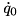frame is located on thebody. If resolveAllInRootFrame is true, all of these frames have their origins resolved in the global frame and their orientation set to match that tof the global frame. constraintForcesOutput contains the wrenches that the constraint applies to the local frames listed in constraintBodyFramesOutput. Note theforce is resolved in theframe.If resolveAllInRootFrame is true, this wrench should be rotated into the coordinates of the root frame. cache a ConstraintCache object which contains ample pre-allocated memory that can be used to reduce the memory footprint of each Constraint implementation. resolveAllInRootFrame When this parameter is - false: the wrenches are resolved into their local frames. - true: the wrenches are resolved into coordinates of the global frame  updateKinematics setting this flag to true will cause all calls to kinematic dependent functions to be updated using the generalized coordinates passed into this function.

Implements Constraint.

Definition at line 176 of file Constraint_Contact.cc.

## ◆ calcConstraintJacobian()

 void calcConstraintJacobian ( Model & model, const double time, const Math::VectorNd & Q, const Math::VectorNd & QDot, Math::MatrixNd & GSysOutput, ConstraintCache & cache, bool updateKinematics = false )
overridevirtual

In this function the matrixof this contraint is inserted into the system constraint Jacobian(GSysOutput). The submatrixbegins at rowInSystem, has sizeOfConstraint number of rows, and fills the full number of columns in G.

Parameters
 model a reference to the multibody model. time the time which is included so that rheonomic constraints might be included (in the future). Q the vector of generalized positions. QDot the vector of generalized velocities. GSysOutput a reference to the constraint Jacobian for the entire system. Insert the G sub-matrix for this constraint beginning and rowInSystem, with a length sizeOfConstraint rows, and a width of the full number of columns in GSysOutput. cache a ConstraintCache object which contains ample pre-allocated memory that can be used to reduce the memory footprint of each Constraint implementation. updateKinematics setting this flag to true will cause all calls to kinematic dependent functions to be updated using the generalized coordinates passed into this function.

Implements Constraint.

Definition at line 89 of file Constraint_Contact.cc.

## ◆ calcGamma()

 void calcGamma ( Model & model, const double time, const Math::VectorNd & Q, const Math::VectorNd & QDot, const Math::MatrixNd & GSys, Math::VectorNd & gammaSysOutput, ConstraintCache & cache, bool updateKinematics = false )
overridevirtual

In this function the vectorof this constraint is inserted into the right-hand-side vectorof the system. The vectorbegins at rowInSystem, and has sizeOfConstraint rows.

Parameters
 model a reference to the multibody model. time the time which is included so that rheonomic constraints might be included (in the future). Q the vector of generalized positions. QDot the vector of generalized velocities. GSys a reference to the constraint Jacobian for the entire system. Ifis needed in this function do not re-evaluate it but instead extract it from the system. gammaSysOutput the system's gamma vector. Insert the gamma sub-vector for this constraint beginning and rowInSystem and with a length of sizeOfConstraint rows. cache a ConstraintCache object which contains ample pre-allocated memory that can be used to reduce the memory footprint of each Constraint implementation. updateKinematics setting this flag to true will cause all calls to kinematic dependent functions to be updated using the generalized coordinates passed into this function.

Implements Constraint.

Definition at line 109 of file Constraint_Contact.cc.

## ◆ calcPointAccelerationError()

 void calcPointAccelerationError ( const std::vector< Math::Vector3d > & pointAccelerationsSys, Math::VectorNd & ddErrSysUpd )

Definition at line 288 of file Constraint_Contact.cc.

## ◆ calcPointAccelerations() [1/2]

 void calcPointAccelerations ( Model & model, const Math::VectorNd & Q, const Math::VectorNd & QDot, const Math::VectorNd & QDDot, Math::Vector3d & pointAccelerationUpd, bool updateKinematics = false )

Definition at line 273 of file Constraint_Contact.cc.

## ◆ calcPointAccelerations() [2/2]

 void calcPointAccelerations ( Model & model, const Math::VectorNd & Q, const Math::VectorNd & QDot, const Math::VectorNd & QDDot, std::vector< Math::Vector3d > & pointAccelerationSysUpd, bool updateKinematics = false )

Definition at line 254 of file Constraint_Contact.cc.

## ◆ calcPointForceJacobian()

 void calcPointForceJacobian ( Model & model, const Math::VectorNd & Q, ConstraintCache & cache, std::vector< Math::SpatialVector > & fExtSysUpd, bool updateKinematics = false )

## ◆ calcPositionError()

 void calcPositionError ( Model & model, const double time, const Math::VectorNd & Q, Math::VectorNd & errSysOutput, ConstraintCache & cache, bool updateKinematics = false )
overridevirtual

In this function the sub vectorof this constraint is inserted into the position error vector of the system. If the constraint is velocity-level constraint or higher (noted in the boolean member variable positionConstraint) this should be set to zero. The vectorbegins at rowInSystem, and has sizeOfConstraint rows.

Parameters
 model a reference to the multibody model time the time which is included so that rheonomic constraints might be included (in the future). Q the vector of generalized positions. errSysOutput the system's constraint position error vector. Insert the position error sub-vector for this constraint beginning and rowInSystem and with a length of sizeOfConstraint rows. cache a ConstraintCache object which contains ample pre-allocated memory that can be used to reduce the memory footprint of each Constraint implementation. updateKinematics setting this flag to true will cause all calls to kinematic dependent functions to be updated using the generalized coordinates passed into this function.

Implements Constraint.

Definition at line 134 of file Constraint_Contact.cc.

## ◆ calcVelocityError()

 void calcVelocityError ( Model & model, const double time, const Math::VectorNd & Q, const Math::VectorNd & QDot, const Math::MatrixNd & GSys, Math::VectorNd & derrSysOutput, ConstraintCache & cache, bool updateKinematics = false )
overridevirtual

In this function the sub vectorof this constraint is inserted into the position error vector of the system. If the constraint is an acceleration-level constraint (noted in the boolean member variable velocityConstraint) this sub vector should be zero. The vectorbegins at rowInSystem, and has sizeOfConstraint rows.

Parameters
 model a reference to the multibody model. time the time which is included so that rheonomic constraints might be included (in the future). Q the vector of generalized positions. QDot the vector of generalized velocities. GSys a reference to the constraint Jacobian for the entire system. Ifis needed in this function do not re-evaluate it but instead extract it from the system: this constraint's sub-matrix begins at rowInSystem, has sizeOfConstraint rows, and the full number of columns of GSys. derrSysOutput the system's constraint velocity error vector. Insert the velocity error sub-vector for this constraint beginning and rowInSystem and with a length of sizeOfConstraint rows. cache a ConstraintCache object which contains ample pre-allocated memory that can be used to reduce the memory footprint of each Constraint implementation. updateKinematics setting this flag to true will cause all calls to kinematic dependent functions to be updated using the generalized coordinates passed into this function.

Implements Constraint.

Definition at line 154 of file Constraint_Contact.cc.

## ◆ getConstraintNormalVectors()

 const std::vector< Math::Vector3d > & getConstraintNormalVectors ( )
inline
Returns
the vector of constraint normals which are resolved in the coordinate system of the ground or base frame.

Definition at line 191 of file Constraint_Contact.h.

## ◆ dblA

 double dblA
private

A working double.

Definition at line 272 of file Constraint_Contact.h.

## ◆ groundPoint

 Math::Vector3d groundPoint
private

The location of the ground reference point.

Definition at line 270 of file Constraint_Contact.h.

## ◆ T

 std::vector< Math::Vector3d > T
private

A vector of the ground normal vectors used in this constraint.

Definition at line 268 of file Constraint_Contact.h.

The documentation for this class was generated from the following files: